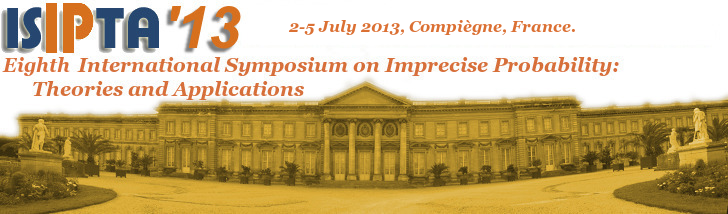Latest info: Pictures now online.

Call for papers and posters as pdf-file.

## CALL FOR PAPERS AND POSTERS

The ISIPTA meetings are the primary international forum to present and discuss new results on the theories and applications of imprecise probability. Imprecise probability is a generic term for the many mathematical and statistical models and methods, allowing us to measure chance or uncertainty without the restriction of sharp probabilities. These models include lower and upper expectations or previsions, interval-valued probabilities, sets of probability measures, belief functions, Choquet capacities, comparative probability orderings, possibility measures, plausibility measures, and sets of desirable gambles. Imprecise probability models are needed in inference and decision problems where the relevant information is scarce, vague or conflicting, and where preferences may be incomplete.

### Symposium format

It is a tradition of the ISIPTA meetings that we try to avoid parallel sessions. Each accepted paper is to be presented both

1. in a plenary session, where we ask for a short introduction and sketch of the context and relevance of the paper; and
2. in a poster session, where ample opportunity and time is given for detailed explanation and discussion.

After the conference, selected papers will appear in a special issue of the International Journal of Approximate Reasoning.

We also invite posters without a paper and hope to attract people who wish to present and discuss their work within the framework of the conference, for instance, for practitioners who wish to discuss possibilities for applications in their field using imprecise probabilities, or for starting students. A poster without paper can also be an option to advertise ongoing work or to discuss challenges.

### Themes of the symposium

The symposium is open to contributions on all aspects of imprecise probability. Topics of interest include, but are not limited to:

• models of coherent imprecise assessments
• sets of probability measures, credal sets
• interval-valued probabilities
• upper and lower expectations or previsions
• non-additive set functions, and in particular Choquet capacities (and Choquet integration), possibility measures, belief and plausibility measures
• random sets
• rough sets
• comparative probability orderings
• imprecision in utilities and expected utilities
• limit laws for imprecise probabilities
• physical models of imprecise probability
• philosophical foundations for imprecise probabilities
• psychological models for imprecision and indeterminacy in probability assessments
• elicitation techniques for imprecise probabilities
• robust statistics
• probabilistic bounding analysis
• data mining with imprecise probabilities
• dealing with missing data
• estimation and learning of imprecise probability models
• decision making with imprecise probabilities
• ambiguity aversion and economic models of imprecise probability
• uncertainty in financial markets
• game-theoretic probability
• algorithms for manipulating imprecise probabilities
• Dempster-Shafer theory
• information algebras and probabilistic argumentation systems
• probabilistic logic, propositional and first-order
• credal networks and other graphical models
• credal classification
• applications in engineering, computer science and artificial intelligence, statistics, economics, finance, management, psychology, philosophy and related fields.

### Submissions

All papers will be reviewed. Accepted papers will be published online and in print in the Conference Proceedings. If you wish to present a poster without paper, please submit a one-page abstract of the work you intend to present. Abstracts of accepted posters will be made available at the conference and online.

Send any remarks to isipta13@hds.utc.fr.